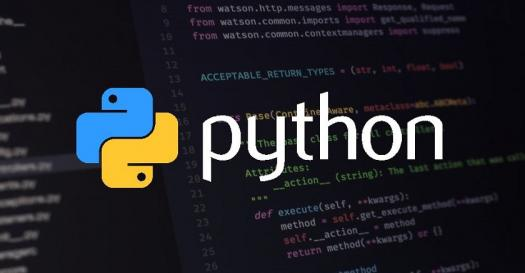# Python Introduction Quiz

10 Questions | Total Attempts: 2083SettingsCreate your own QuizPython is a high-level programming language. Play this skill testing quiz on "Python Introduction" and gauge your python skill level. This mainly introductory questions that will help you assess your competency and skills in this language. It has easy, moderate, and high-level questions that are certainly going to provide you with a valuable addition to your existing knowledge. Attempt this to get an edge over your peers and harness industry-relevant knowledge. If you like the quiz, share it with your friends and family. All the best!

• 1.
Which of the following statements is true?
• A.

Python is a high level programming language

• B.

Python is an interpreted language

• C.

Python is an object-oriented language

• D.

All of the above

• 2.
What is used to define a block of code (body of loop, function etc.) in Python?
• A.

Curly braces

• B.

Parenthesis

• C.

Indentation

• D.

Quotation

• 3.
Which of the following is correct?
• A.

Comments are for programmers for better understanding of the program

• B.

Python Interpreter ignores comment

• C.

You can write multi-line comments in Python using triple quotes, either ''or "

• D.

All of the above

• 4.
Which of the following is correct?
• A.

Variable name can start with an underscore

• B.

Variable name can start with a digit

• C.

Keywords cannot be used as a variable name.

• D.

Variable name can have symbols like: @, #, \$ etc

• 5.
In the following code, n is a/an _______? n = '5'
• A.

Integer

• B.

String

• C.

Tuple

• D.

Operator

• 6.
What is the output of the following code? print(1, 2, 3, 4, sep='*')
• A.

1 2 3 4

• B.

1234

• C.

1*2*3*4

• D.

24

• 7.
What is used to take input from the user in Python?
• A.

Vin

• B.

Scanf()

• C.

Input()

• D.

<>

• 8.
What is the output of the following code? numbers = [2, 3, 4] print(numbers)
• A.

2, 3, 4

• B.

2 3 4

• C.

[2, 3, 4]

• D.

[1 2 3]

• 9.
What is the output of the following code? print(3 >= 3)
• A.

3 >= 3

• B.

True

• C.

False

• D.

None

• 10.
The statement using and operator results true if _______
• A.

Both operands are true

• B.

Both operands are false

• C.

Either of the operands is true

• D.

First operand is trueBack to top
×

Wait!
Here's an interesting quiz for you.